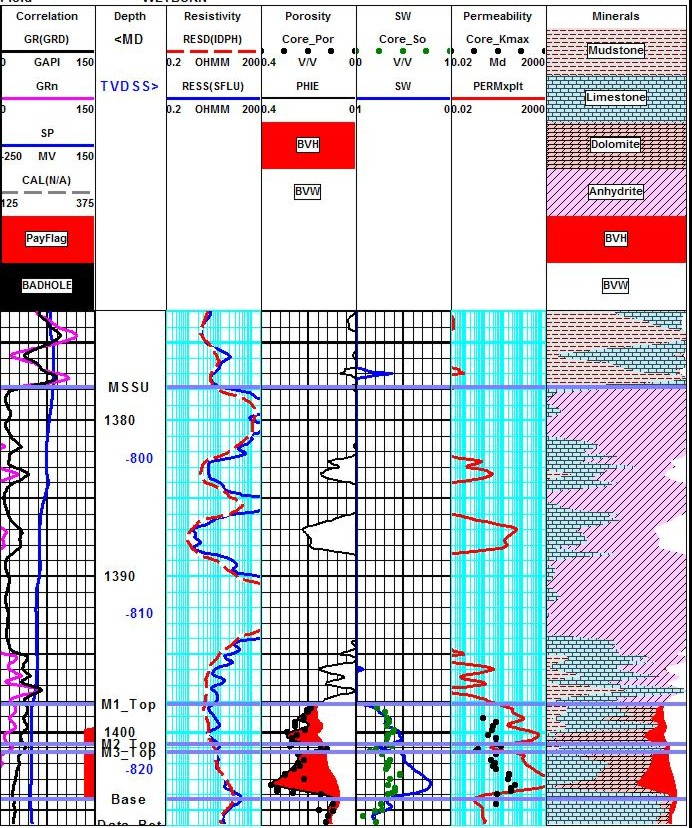Moveable Hydrocarbon Saturation
Since water saturation is the natural result of the previous calculations, it is often reported by the log analyst as one of "the answers". However, it is the amount of oil or gas, not water, that is wanted. This information flows from the following equations.

Total hydrocarbon saturation:
1: Shy = 1.00 - Sw

Residual hydrocarbon saturation:
2: Shr = 1.00 - Sxo

Moveable hydrocarbon saturation:
3: Smo = Shy - Shr
OR 3A: Smo = Sxo - Sw

Recovery Factor:
4: RF = Shr / Shy

Where:
Sxo = water saturation in invaded zone (fractional)
Sw = water saturation in un-invaded zone (fractional)
Shy = hydrocarbon saturation in un-invaded zone (fractional)
Shr = residual hydrocarbon saturation in invaded zone (fractional)
Smo = moveable hydrocarbon saturation (fractional)COMMENTS:
These saturations are needed in order to find hydrocarbon volume which could be produced from the well.

CAUTION: There are many situations where Smo cannot be calculated, and many silly results have been presented to clients over the years. If invasion is very shallow, Smo may be zero when in fact the zone is an excellent producer. Likewise, deep invasion gives useless results. If your computer program insists on calculating Smo, you will have to fiddle with RMF to get rational results, or set RMF > 10 to make Sxo = 1.0 always.Fluid Volume Calculations
For reserves calculations, it is sometimes convenient to convert saturations to volume of fluid per unit volume of rock. These volumes are often called bulk volume and the "per unit rock volume" is unstated. These volumes are also needed when using computers for plotting log analysis results versus depth.

Water volume:
5: Vwatr = PHIe * Sw

Total hydrocarbon volume:
6: Vhydt = PHIe * Shy

Moveable hydrocarbon volume:
7: Vhydm = PHIe * Smo

Residual hydrocarbon volume:
8: Vhydr = PHIe * Shr

Rock matrix volume:
9: Vrock = 1.00 - PHIe - Vsh

Where:
PHIe = porosity from any method (fractional)
Shr = residual hydrocarbon saturation in invaded zone (fractional)
Shy = hydrocarbon saturation in un-invaded zone (fractional)
Smo = moveable hydrocarbon saturation (fractional)
Sw = water saturation in un-invaded zone (fractional)
Vrock = volume of matrix (fractional)
Vsh = volume of shale (shale content) (fractional)
Vhydt = bulk volume hydrocarbon (fractional)
Vhydm = bulk volume moveable (fractional)
Vhydr = bulk volume residual (fractional)
Vwatr = bulk volume water (fractional)COMMENTS:
This data is used to calculate hydrocarbons in place and recoverable reserves.

When making depth plots of log analysis results, the usual approach is to plot the following curves on a scale of zero to one:

Value to Plot                                   Result
Vwatr                                               water volume
Vwatr + Vhydm                                moved hydrocarbon
Vwatr + Vhydm + Vhydr                  residual hydrocarbon
Vwatr + Vhydm + Vhydr + Vrock     matrix rock plus porosity

The remaining distance to the border of the track will be Vsh, the shale volume. If bound water volume is desired on the plot, it can be presented as part of the shale volume by plotting;
Vwatr + Vhydm + Vhydr + Vrock + (Vsh * BVWSH)

If the rock volume is broken into two or more constituents then these additional curves should be plotted and shaded appropriately.Moveable Hydrocarbon EXAMPLE
This example shows a comparison of residual oil from core in a depleted zone (M1 interval) and in a bypassed zone (M3 interval). The Sor from core equals (1 - SW) from log analysis, so there is no moveable oil in the M1. The close match suggests that most of the saturation parameters (A, M, N, RW@FT) and porosity are reasonably well calibrated. Since the world abounds with depleted zones (most are well known to the well operators) this test should always be made to confirm SW parameters where ever core data is available.Computed results for carbonate example. Note higher water saturation on M1 compared to M3. M3 is bypassed pay. M1 is depleted oil. Dots are core data. Note that residual oil on core in M1 matches calculated
Sor = (1 - SW). In M3, Sor on core is less than (1 - SW) from log analysis, so there is moveable oil in
M3 interval. Calibration to core permeability needs more work to get a decent match.

Page Views ---- Since 01 Jan 2015